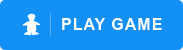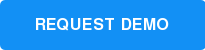# Play ST Math Games

PreK-1
Match Count

### Topic: Counting, Cardinality

Students are introduced to counting and numbers by choosing the number of circles blocking JiJi, the penguin, from crossing the path. Puzzles begin with all visual questions that require students to use 1:1 correspondence to match the quantity given. As the puzzles transition, students have to represent numerals with the corresponding quantity of circles.

As the puzzles transition, students solve multi-step puzzles to determine the height JiJi will end up at.PreK-1
Match Shape

### Topic: Geometry

Help JiJi, the penguin, walk across the road! Students have to match the shape in JiJi’s path from a set of shapes in outline. Students attend to the attributes of two-dimensional shapes and develop a strategy to eliminate shapes that do not match and identify the shape that does.

As the puzzles transition, more shapes are given and eventually shape names provide students with an opportunity to use language labels to describe given shapes.K-2
Push Box

Students are introduced to the concept of addition from 1 to 10 by selecting the number of blocks needed to get JiJi, the penguin, to the height of the platform. By visually introducing addition as stacking blocks and subtraction as holes in the ground, students see how addition and subtraction are the inverse of each other.

As the puzzles transition, students solve multi-step puzzles to determine the height JiJi will end up at.K-5
Upright JiJi

### Topic: Spatial Reasoning

Can you rotate JiJi, the penguin, into an upright position?

This task specifically targets students' spatial temporal reasoning ability as they manipulate 3D JiJi into an upright position.

Upright JiJi develops pre-algebraic thinking and reasoning, as students select the correct set of operators to transform the input into the output.3-5
Leg Drape

### Topic: Multiplication, Division

How many shoes are needed for all the animals feet?

Students develop their understanding of equal groups multiplication, through 40, by selecting the number of shoes required by the group of animals.

In later levels, the game uses the same model to represent division and multi-step problem situations, building a robust and interconnected schema.3-5
Fair Sharing

### Topic: Division

Drag blocks onto creatures in order to divide a set of blocks into equal piles. Through this task, students model the task of "fair sharing" division.

Students will slowly transition to answering "fair sharing" questions up to 40 in a more symbolic representation, building their division schema on a conceptual foundation.3-5
JiJi Cycle

### Topic: Fractions

What is the relationship between fraction pies and the number line?

In this creative model, students estimate how far a set of fraction wedges will roll JiJi onto the number line.

As the level progresses, students must weigh how the impact of unit-fractions, fraction addition, and subtraction will alter the solution.3-6
Volume Conversion

### Topic: Conversion

Students use visual models to build their understanding of measurements of capacity. As they play through the puzzles students experience problems requiring them to convert between cups, pints, quarts and gallons.3, 5-8
Volume Fill

### Topic: Volume

Can you find the correct volume in the cylinder to fill the blocks perfectly?

Volume fill builds students conceptual understanding of volume as not just a formula to be memorized, but a measure of a finite amount of 3D space. As the levels progress, the shapes become irregular - further challenging students and deepening understanding.5-8
Wall Factory

### Topic: Expressions, Variables

Identify the expression that matches the pattern of blocks in the ground and enter the unknown values to help JiJi cross the screen. Students develop understanding of the meaning of variables, expressions, and the order of operations.

Puzzles transition from simple one step expressions where students find the value of the unknown to multi step problems where they apply order of operations to match a visual pattern.